Next: 3.4 Monte Carlo Method Up: 3.3 Master Equation Method Previous: 3.3 Master Equation Method

## 3.3.1 Krylov Subspace Approximate of the Matrix Exponential Operator

Decomposing a matrix X into X=SBS-1makes it possible to write the matrix exponential of X as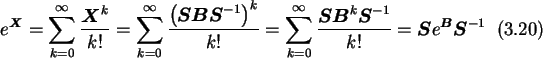A particular decomposition is the  Jordan canonical form which is for a matrix X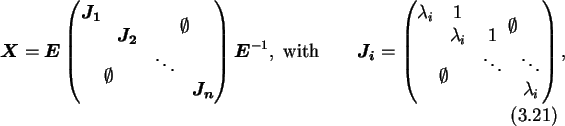where Ji are the Jordan blocks and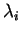are the eigenvalues of X. A Jordan block Ji has typically a dimension of one. This is the case if the algebraic multiplicity and the geometric multiplicity ofare equal. Otherwise the dimension of the Jordan block increases by the difference of algebraic to geometric multiplicity. If all Jordan blocks have dimension one the matrix is said to be non-defective or diagonalizable. Using the Jordan canonical form the    exponential of Xt is given by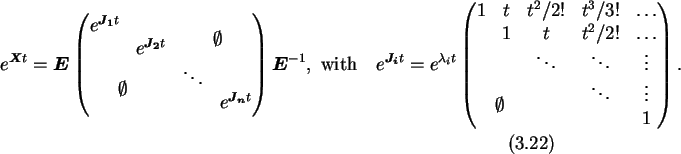One needs to calculate the exponential of the transition rate matrix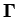(see(3.19)). Due to the special structure ofwith main diagonal elements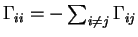it can be shown by Gershgorin's circle theorem that all its eigenvalues lie in the left half-plane and one eigenvalue is zero (see left side of Fig. 3.8).It is known that Krylov subspace methods tend to provide good approximations for the eigenvalues located in the outermost part of the spectrum . Since all eigenvalues oflie in the left half-plane, the eigenvalues which dominate the exponential function,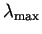, are located in the inner part of the spectrum. Thus the eigenvalue spectrum ofhas to be transformed to make the eigenvalues which dominate the exponential function coincide with the eigenvalues which are filtered out by the Krylov subspace method. An inversion is not possible sinceis singular. One can move the eigenvalue spectrum to the right with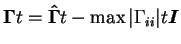(see right side of Fig. 3.8). In addition the upper limit of the spectral radius derived from Gershgorin's disc theorem is reduced by a factor of two. Since the commutative law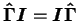applies, one may write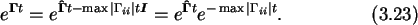The transformed matrix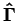has its eigenvalues which dominate the exponential function in the outermost part of its spectrum. Thus, a Krylov subspace method will give good results even for small dimension subspaces.

The objective is the computation of an approximation of the form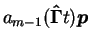to the matrix exponential operation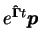, where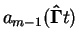is a polynomial of degree m-1 in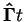, which is a linear combination of the vectors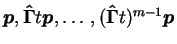, and thus is an element of the Krylov subspace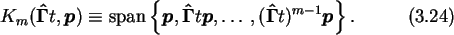Constructing an  orthonormal basis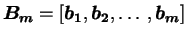in the Krylov subspace, and choosing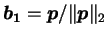, one may write using the identity B
mBmT=I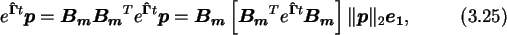where e
1 is the unit vector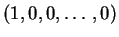. The purpose of the Krylov subspace approach, namely to project the exponential of a large matrix approximately onto a small Krylov subspace, is accomplished by approximating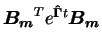with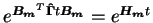. This gives the approximation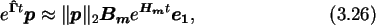which still involves the evaluation of the exponential of a matrix, but this time of small dimension m and of a particular structure, namely upper Hessenberg. B
m and Hm can be computed by the Arnoldi algorithm (see Appendix H), which is a modified Gram-Schmidt orthogonalization. Y. Saad  shows that an a priori error bound exists.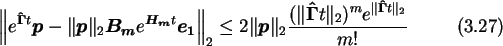Next: 3.4 Monte Carlo Method Up: 3.3 Master Equation Method Previous: 3.3 Master Equation Method

Christoph Wasshuber